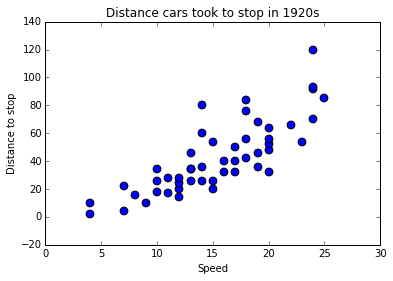# Scatter plot in Python¶

First we will need a dataset, let us learn to use rdatatset in Python https://vincentarelbundock.github.io/Rdatasets/datasets.html

In :
# import the packages required

import numpy as np
import scipy as sc
import statsmodels.api as sm
import matplotlib.pyplot as plt

In :
# import the data set using statsmodel.api
cars_speed=sm.datasets.get_rdataset("cars", "datasets")


Each dataset in rdataset has a description attached to it, contained in the doc file on the website. This document can be printed using python docstrings

In :
print cars_speed.__doc__

+--------+-------------------+
| cars   | R Documentation   |
+--------+-------------------+

Speed and Stopping Distances of Cars
------------------------------------

Description
~~~~~~~~~~~

The data give the speed of cars and the distances taken to stop. Note
that the data were recorded in the 1920s.

Usage
~~~~~

::

cars

Format
~~~~~~

A data frame with 50 observations on 2 variables.

+--------+---------+-----------+--------------------------+
| [,1]   | speed   | numeric   | Speed (mph)              |
+--------+---------+-----------+--------------------------+
| [,2]   | dist    | numeric   | Stopping distance (ft)   |
+--------+---------+-----------+--------------------------+

Source
~~~~~~

Ezekiel, M. (1930) *Methods of Correlation Analysis*. Wiley.

References
~~~~~~~~~~

McNeil, D. R. (1977) *Interactive Data Analysis*. Wiley.

Examples
~~~~~~~~

::

require(stats); require(graphics)
plot(cars, xlab = "Speed (mph)", ylab = "Stopping distance (ft)",
las = 1)
lines(lowess(cars$speed, cars$dist, f = 2/3, iter = 3), col = "red")
title(main = "cars data")
plot(cars, xlab = "Speed (mph)", ylab = "Stopping distance (ft)",
las = 1, log = "xy")
title(main = "cars data (logarithmic scales)")
lines(lowess(cars$speed, cars$dist, f = 2/3, iter = 3), col = "red")
summary(fm1 <- lm(log(dist) ~ log(speed), data = cars))
opar <- par(mfrow = c(2, 2), oma = c(0, 0, 1.1, 0),
mar = c(4.1, 4.1, 2.1, 1.1))
plot(fm1)
par(opar)

## An example of polynomial regression
plot(cars, xlab = "Speed (mph)", ylab = "Stopping distance (ft)",
las = 1, xlim = c(0, 25))
d <- seq(0, 25, length.out = 200)
for(degree in 1:4) {
fm <- lm(dist ~ poly(speed, degree), data = cars)
assign(paste("cars", degree, sep = "."), fm)
lines(d, predict(fm, data.frame(speed = d)), col = degree)
}
anova(cars.1, cars.2, cars.3, cars.4)



Next let us print the data to see its content.

In :
print cars_speed.data

    speed  dist
0       4     2
1       4    10
2       7     4
3       7    22
4       8    16
5       9    10
6      10    18
7      10    26
8      10    34
9      11    17
10     11    28
11     12    14
12     12    20
13     12    24
14     12    28
15     13    26
16     13    34
17     13    34
18     13    46
19     14    26
20     14    36
21     14    60
22     14    80
23     15    20
24     15    26
25     15    54
26     16    32
27     16    40
28     17    32
29     17    40
30     17    50
31     18    42
32     18    56
33     18    76
34     18    84
35     19    36
36     19    46
37     19    68
38     20    32
39     20    48
40     20    52
41     20    56
42     20    64
43     22    66
44     23    54
45     24    70
46     24    92
47     24    93
48     24   120
49     25    85


We can access the columns of this data set in the following way:

In :
print cars_speed.data['speed']

0      4
1      4
2      7
3      7
4      8
5      9
6     10
7     10
8     10
9     11
10    11
11    12
12    12
13    12
14    12
15    13
16    13
17    13
18    13
19    14
20    14
21    14
22    14
23    15
24    15
25    15
26    16
27    16
28    17
29    17
30    17
31    18
32    18
33    18
34    18
35    19
36    19
37    19
38    20
39    20
40    20
41    20
42    20
43    22
44    23
45    24
46    24
47    24
48    24
49    25
Name: speed, dtype: int64

In :
print cars_speed.data['dist']

0       2
1      10
2       4
3      22
4      16
5      10
6      18
7      26
8      34
9      17
10     28
11     14
12     20
13     24
14     28
15     26
16     34
17     34
18     46
19     26
20     36
21     60
22     80
23     20
24     26
25     54
26     32
27     40
28     32
29     40
30     50
31     42
32     56
33     76
34     84
35     36
36     46
37     68
38     32
39     48
40     52
41     56
42     64
43     66
44     54
45     70
46     92
47     93
48    120
49     85
Name: dist, dtype: int64


Next we will plot a scatter plot between these variables using matplotlib

In :
#make matplotlib inline
%matplotlib inline

#scatter plot
plt.scatter(cars_speed.data['speed'],cars_speed.data['dist'],c='b',s=60)

# xlable of the scatter plot
plt.xlabel('Speed')

# ylabel of the scatter plot
plt.ylabel('Distance to stop')

# title of the scatter plot
plt.title('Distance cars took to stop in 1920s')

Out:
<matplotlib.text.Text at 0x101a8f190>In [ ]: